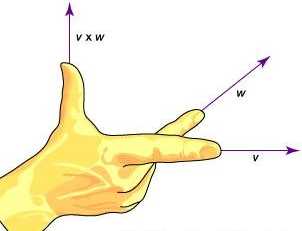Courses

# Vectors, Newton's law of Motion Physics Notes | EduRev

## Physics : Vectors, Newton's law of Motion Physics Notes | EduRev

The document Vectors, Newton's law of Motion Physics Notes | EduRev is a part of the Physics Course Basic Physics for IIT JAM.
All you need of Physics at this link: Physics

Vector, in mathematics, a quantity that has both magnitude and direction but not position. Examples of such quantities are velocity and acceleration. In their modern form, vectors appeared late in the 19th century when Josiah Willard Gibbs and Oliver Heaviside (of the United States and Britain, respectively) independently developed vector analysis to express the new laws of electromagnetism discovered by the Scottish physicist James Clerk Maxwell. Since that time, vectors have become essential in physicsmechanics, electrical engineering, and other sciences to describe forces mathematically.

Vectors may be visualized as directed line segments whose lengths are their magnitudes. Since only the magnitude and direction of a vector matter, any directed segment may be replaced by one of the same length and direction but beginning at another point, such as the origin of a coordinate system. Vectors are usually indicated by a boldface letter, such as v. A vector’s magnitude, or length, is indicated by |v|, or v, which represents a one-dimensional quantity (such as an ordinary number) known as a scalar. Multiplying a vector by a scalar changes the vector’s length but not its direction, except that multiplying by a negative number will reverse the direction of the vector’s arrow. For example, multiplying a vector by 1/2 will result in a vector half as long in the same direction, while multiplying a vector by −2 will result in a vector twice as long but pointed in the opposite direction.

Two vectors can be added or subtracted. For example, to add or subtract vectors v and graphically, move each to the origin and complete the parallelogram formed by the two vectors; v + w is then one diagonal vector of the parallelogram, and vw is the other diagonal vector.

There are two different ways of multiplying two vectors together. The cross, or vector, product results in another vector that is denoted by v × w. The cross product magnitude is given by |v × w| = vw sin θ, where θ is the smaller angle between the vectors (with their “tails” placed together). The direction of v × w is perpendicular to both v and w, and its direction can be visualized with the right-hand rule, as shown in the figure. The cross product is frequently used to obtain a “normal” (a line perpendicular) to a surface at some point, and it occurs in the calculation of torque and the magnetic force on a moving charged particle.Right hand rule

The ordinary, or dot, product of two vectors is simply a one-dimensional number, or scalar. In contrast, the cross product of two vectors results in another vector whose direction is orthogonal to both of the original vectors, as illustrated by the right hand rule. The magnitude, or length, of the cross product vector is given by v,w sin θ, where θ is the angle between the original vectors v and w.

The other way of multiplying two vectors together is called a dot product, or sometimes a scalar product because it results in a scalar. The dot product is given by v ∙ w = vw cos θ, where θ is the smaller angle between the vectors. The dot product is used to find the angle between two vectors. (Note that the dot product is zero when the vectors are perpendicular.) A typical physical application is to find the work W performed by a constant force F acting on a moving object d; the work is given by W = Fd cos θ.

Offer running on EduRev: Apply code STAYHOME200 to get INR 200 off on our premium plan EduRev Infinity!

## Basic Physics for IIT JAM

77 videos|156 docs|94 tests

,

,

,

,

,

,

,

,

,

,

,

,

,

,

,

,

,

,

,

,

,

,

,

,

;